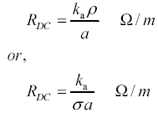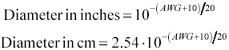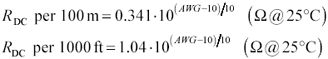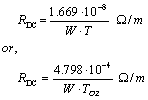# DC Resistance

Practical transmission lines  incorporate series resistance. The nonzero resistance of practical transmission lines dissipates a portion of the signal power, causing both attenuation (loss) and distortion in propagating signals. This section shows how to estimate the DC resistance (per meter) of a transmission line.

 Thin-film superconducting transmission lines have essentially no series resistance provided that you keep them cold. For high-speed digital applications the requirement for cold-temperature operation renders them, in this author's opinion, not practical.

Equation 2.37where a is the cross-sectional area of the conductor (m 2 ), k a is a correction factor that accounts for the additional DC resistance of the return path , r is the resistivity of the conductor ( W “m), or s is the conductivity of the conductor (S/m).

For annealed copper at room temperature r = 1.724 ·10 “8 W “m and s = 5.800 ·10 7 S/m.

Note that the effective DC resistance of a transmission-line structure is the sum of the DC resistance of the signal conductor plus the DC resistance of the return path. In [2.37] the factor 1/ s a accounts only for the resistance of the signal conductor. The factor k a accounts for the additional resistance of the return path. The factor k a equals a quotient , the numerator of which is the sum of the DC resistances of the signal and return paths, and the denominator of which is the DC resistance of the signal path alone.

For example, a pcb trace with a wide, flat return path has K a = 1, because the resistance of the wide, flat return path is so low it adds almost nothing to the overall DC resistance of the structure.

A twisted-pair configuration, because the outbound (signal) and return (~signal) wires are the same, has K a = 2.

A coaxial cable has a DC resistance equal to the sum of the resistances of the center conductor and shield. In many cases the center conductor may be composed of plated or clad materials. The shield often incorporates a braided construction with or without an inner foil wrap. When working with coaxial cables it's usually best to just accept the worst-case specification provided by the manufacturer for R DC and not try to compute it.

The series resistance for long cables is often specified in ohms per 1,000 feet or ohms per 100 meters . When dealing with twisted-pair cables, the specified resistance usually includes the series resistance of both the outgoing and return wires. For coaxial cables the manufacturer often separately specifies the series resistances of the center conductor and outer shield. In any calculations of signal loss you must always add the resistance of both outgoing and return pathways , because current flows equally in both, and both dissipate power.

Here are eight rules of thumb for estimating the DC resistance of round copper wires:

1. The American Wire Gauge (AWG) system is a logarithmic measure of the diameter of round wires. The larger the gauge, the smaller the wire.
2. Six AWG points halves the diameter.
3. Area being proportional to diameter-squared, three AWG points halves the cross-sectional area.
4. Three AWG points doubles the wire resistance.
5. A round conductor of size #24 American Wire Gauge (24-AWG) has a nominal diameter of 0.507 mm (0.02 in.) and a resistance at room temperature of 0.085 W /m (26 W /1000 ft).
6. Twisted-pair 24-AWG cable has a total series resistance (adding both wires in series) of 0.170 W /m (52 W /1000 ft) at room temperature.
7. RG-58/U coaxial cable using a stranded core of AWG 20 has a resistance at room temperature of 0.034 W /m (10.3 W /1000 ft).
8. The resistance of copper increases 0.39% with every 1 °C increase in temperature. Over a 70 °C temperature range, that amounts to a variation of 31%.

Here are some handy equations for working with AWG sizes:

Equation 2.38Equation 2.39Equation 2.40The resistance (per unit length) of a printed-circuit trace varies with copper thickness and trace width. The trace thickness is rated in plating weight, typically reported in ounces. A 1-oz plating corresponds to a thickness of 34.8 m m. The thickness scales in proportion to plating weight.  The resistance of an electrodeposited pure copper pcb trace may be calculated from its thickness and width.

 Plating weight in units of ounces refers to the number of ounces of material deposited on a flat surface one foot square.

Equation 2.41where R DC s the series resistance of the line ( W /m), W is the width of line (m), T is the thickness of line (m), which equals 3.48 ·10 “5 meters (1.37 mil) for one ounce of plating weight, or T OZ is the plating weight of the line in ounces (oz/ft 2 ).

A 1/2-oz copper pcb trace with 100- m m (4-mil) width has a DC resistance of 9.6 W /m.

POINT TO REMEMBER

• The nonzero resistance of practical transmission lines dissipates a portion of the signal power, causing both attenuation (loss) and distortion in propagating signals.High-Speed Signal Propagation[c] Advanced Black Magic
ISBN: 013084408X
EAN: N/A
Year: 2005
Pages: 163